# Carnot Cycle State 1 Thot q0 wCV T

• Slides: 26
Download presentation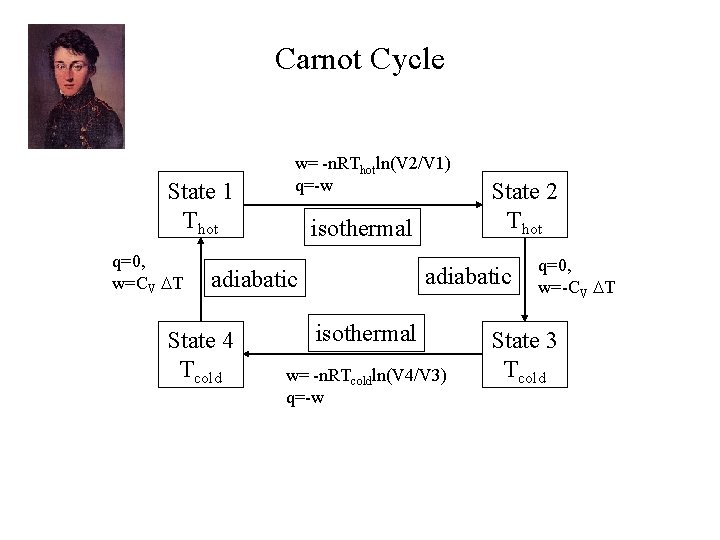Carnot Cycle State 1 Thot q=0, w=CV T w= -n. RThotln(V 2/V 1) q=-w isothermal adiabatic State 4 Tcold State 2 Thot isothermal w= -n. RTcoldln(V 4/V 3) q=-w q=0, w=-CV T State 3 Tcold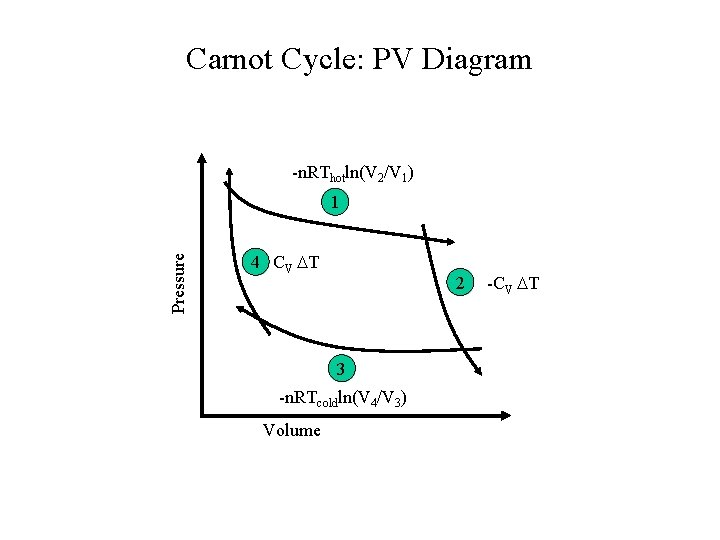Carnot Cycle: PV Diagram -n. RThotln(V 2/V 1) Pressure 1 4 CV T 2 3 -n. RTcoldln(V 4/V 3) Volume -CV TCarnot Cycle, Schematic View Thot E Tcold The engine operates between two reservoirs to and from which heat can be transferred. We put heat into the system from the hot reservoir and heat is expelled into the cold reservoir.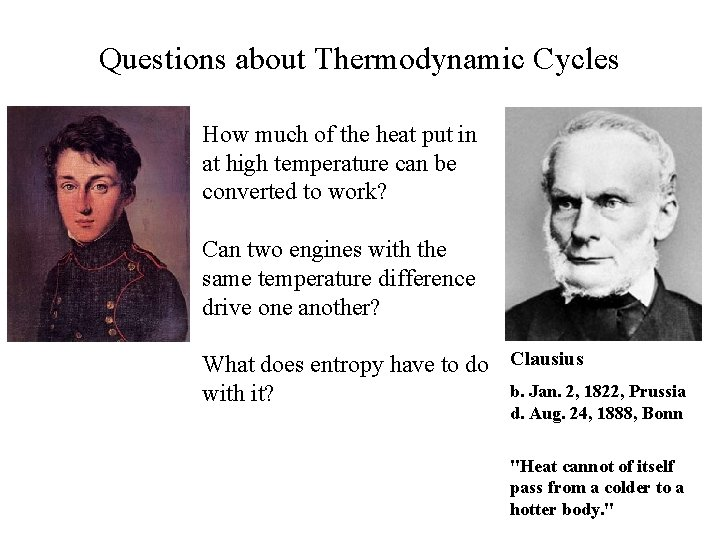Questions about Thermodynamic Cycles How much of the heat put in at high temperature can be converted to work? Can two engines with the same temperature difference drive one another? What does entropy have to do Clausius b. Jan. 2, 1822, Prussia with it? d. Aug. 24, 1888, Bonn "Heat cannot of itself pass from a colder to a hotter body. "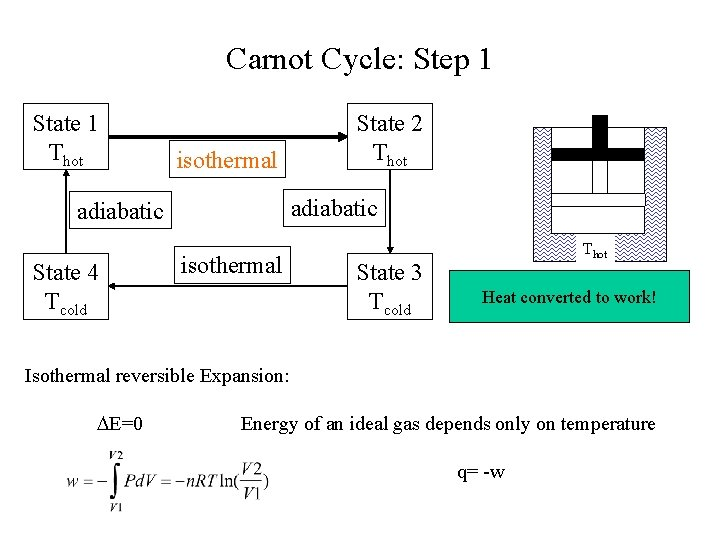Carnot Cycle: Step 1 State 1 Thot isothermal adiabatic State 4 Tcold State 2 Thot isothermal State 3 Tcold Thot Heat converted to work! Isothermal reversible Expansion: E=0 Energy of an ideal gas depends only on temperature q= -w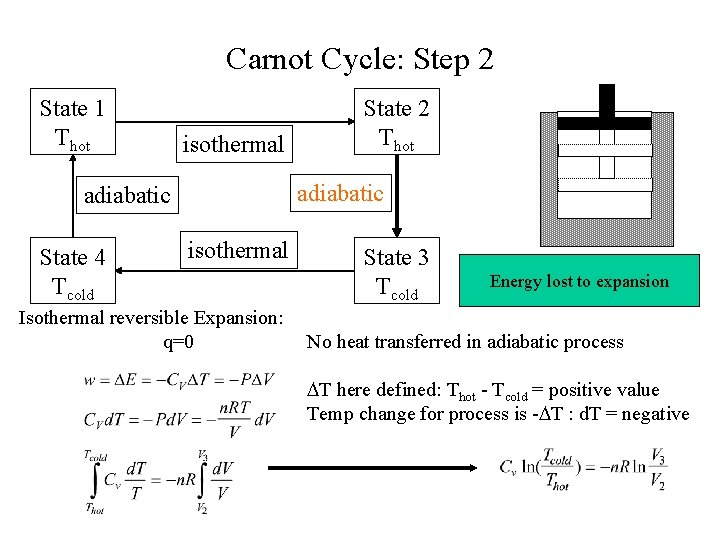Carnot Cycle: Step 2 State 1 Thot isothermal adiabatic State 4 Tcold State 2 Thot isothermal Isothermal reversible Expansion: q=0 State 3 Tcold Energy lost to expansion No heat transferred in adiabatic process T here defined: Thot - Tcold = positive value Temp change for process is - T : d. T = negativeCarnot Cycle: Steps 3 & 4 State 1 Thot isothermal adiabatic State 4 Tcold State 2 Thot isothermal State 3 Tcold Work done in compressionCarnot Cycle: Summary Step 1 Step 2 Step 3 Step 4 w -n. RThotln(V 2/V 1) -CV T -n. RTcoldln(V 4/V 3) CV T q -w 0 E 0 w Thot w 1 + w 2 + w 3 + w 4= -n. RThotln(V 2/V 1) - n. RTcoldln(V 4/V 3) E q 1 + q 2 + q 3 + q 4= n. RThotln(V 2/V 1) + n. RTcoldln(V 4/V 3) q = -w Heat flows through system, some work is extracted. Tcold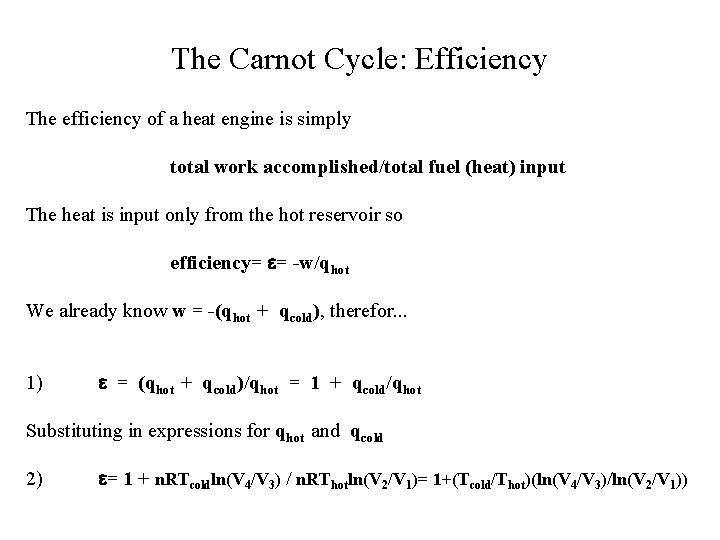The Carnot Cycle: Efficiency The efficiency of a heat engine is simply total work accomplished/total fuel (heat) input The heat is input only from the hot reservoir so efficiency= = -w/qhot We already know w = -(qhot + qcold), therefor. . . 1) = (qhot + qcold)/qhot = 1 + qcold/qhot Substituting in expressions for qhot and qcold 2) = 1 + n. RTcoldln(V 4/V 3) / n. RThotln(V 2/V 1)= 1+(Tcold/Thot)(ln(V 4/V 3)/ln(V 2/V 1))= 1 + n. RTcoldln(V 4/V 3) / n. RThotln(V 2/V 1)= 1+(Tcold/Thot)(ln(V 4/V 3)/ln(V 2/V 1)) This second expression is rather complicated-- but we have a relationship for the volumes in this process: Rearranging and plugging it in to the first equation on the page: = 1 - (Tcold/Thot)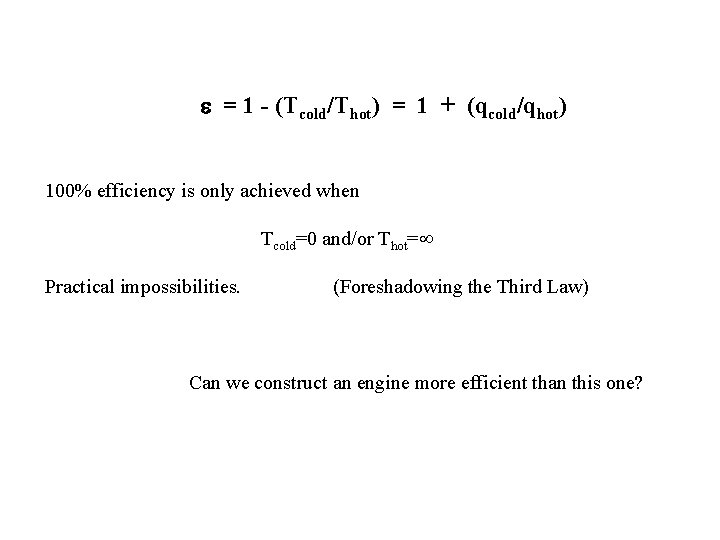= 1 - (Tcold/Thot) = 1 + (qcold/qhot) 100% efficiency is only achieved when Tcold=0 and/or Thot= Practical impossibilities. (Foreshadowing the Third Law) Can we construct an engine more efficient than this one?Impossible Machines Consider two engines ER and E’ operating between the same two reservoirs: Can the efficiencies of these two Thot engines be different? ER E’ We operate ER in reverse. We couple the operation of the two engines. We know: Tcold The composite engine then has a total work W= w’-w. R= qhot + qcold (forward direction) w’= q’hot + q’cold Let’s couple the engines so that work from forward running engine drives the other one backwards with no work on the surroundings: w’ = w. R (remember actual work of reverse process is -w. R)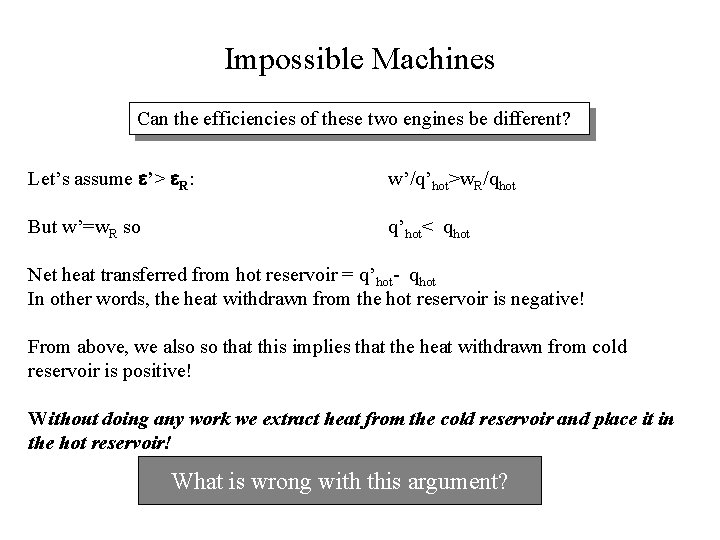Impossible Machines Can the efficiencies of these two engines be different? Let’s assume ’> R: w’/q’hot>w. R/qhot But w’=w. R so q’hot< qhot Net heat transferred from hot reservoir = q’hot- qhot In other words, the heat withdrawn from the hot reservoir is negative! From above, we also so that this implies that the heat withdrawn from cold reservoir is positive! Without doing any work we extract heat from the cold reservoir and place it in the hot reservoir! What is wrong with this argument?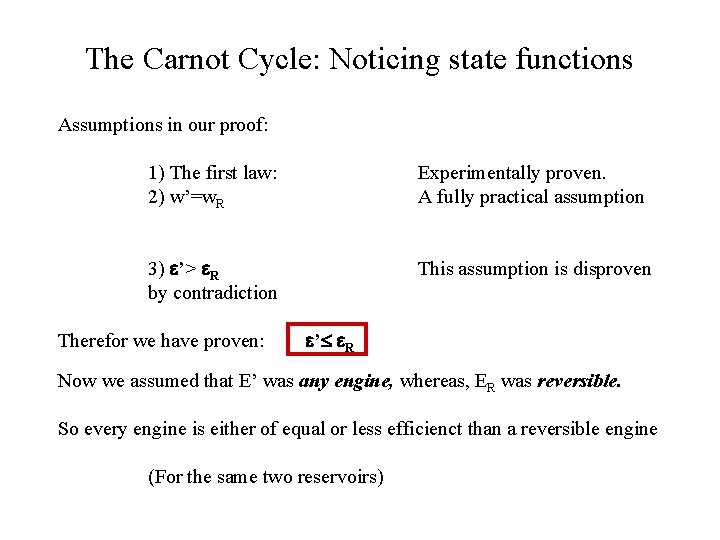The Carnot Cycle: Noticing state functions Assumptions in our proof: 1) The first law: 2) w’=w. R Experimentally proven. A fully practical assumption 3) ’> R by contradiction This assumption is disproven Therefor we have proven: ’ R Now we assumed that E’ was any engine, whereas, ER was reversible. So every engine is either of equal or less efficienct than a reversible engine (For the same two reservoirs)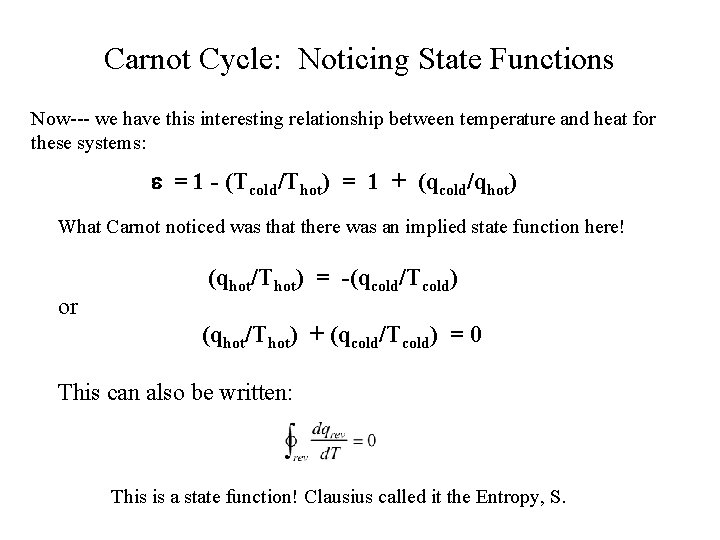Carnot Cycle: Noticing State Functions Now--- we have this interesting relationship between temperature and heat for these systems: = 1 - (Tcold/Thot) = 1 + (qcold/qhot) What Carnot noticed was that there was an implied state function here! or (qhot/Thot) = -(qcold/Tcold) (qhot/Thot) + (qcold/Tcold) = 0 This can also be written: This is a state function! Clausius called it the Entropy, S.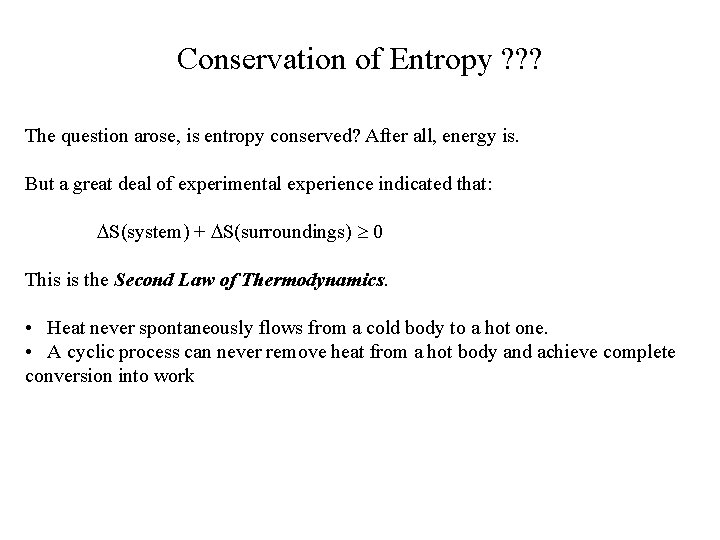Conservation of Entropy ? ? ? The question arose, is entropy conserved? After all, energy is. But a great deal of experimental experience indicated that: S(system) + S(surroundings) 0 This is the Second Law of Thermodynamics. • Heat never spontaneously flows from a cold body to a hot one. • A cyclic process can never remove heat from a hot body and achieve complete conversion into work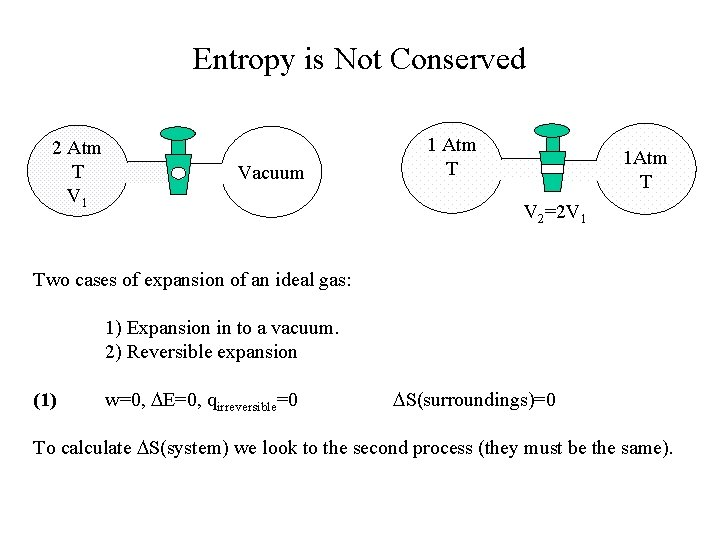Entropy is Not Conserved 2 Atm T V 1 Vacuum 1 Atm T 1 Atm T V 2=2 V 1 Two cases of expansion of an ideal gas: 1) Expansion in to a vacuum. 2) Reversible expansion (1) w=0, E=0, qirreversible=0 S(surroundings)=0 To calculate S(system) we look to the second process (they must be the same).Entropy is Not Conserved 2 Atm T V 1 1 Atm T Vacuum 1 Atm T V 2=2 V 1 In the second process we follow an isothermal, reversible path. We know that T and thus E=0 Now…qrev= E - w= RT ln(2) so S(system)= qrev/T= R ln(2)Entropy is Not Conserved For the reversible process we’ve already calculated qrev= RT ln(V 2/V 1) = RT ln(2) S(system) = qrev/T= R ln(2) One way to make sure this is reversible is to make sure the outside temperature is only differentially hotter. In this case, S(surroundings) = -qrev/T S(total) = 0 For the total irreversible process, S(surroundings) = 0 (nothing exchanged) S (total) = 0 + R ln(2) > 0Dissorder and Entropy It turns out that disorder and entropy are intimately related. Ludwig Boltzmann c. 1875 We start out by considering the spontaneity of this process. Why doesn’t the gas spontaneously reappear back in the box?Dissorder and Entropy Let’s break the box into N cells and consider the gas to be an ideal gas composed of M molecules. * We ask: What is the probability that M molecules will be in the box labeled ‘*’ This obviously depends on both M and N. We assume N>M for this problem. Number of ways of distributing M indistinguishable balls in N boxes is approximately: ~ NM/M! (this approx. miscounts when multiple balls in same box) Boltzmann noted that an entropy could be defined as S= k ln( )= R ln( )/NA There a number of reasons this is a good definition. One is it connects to thermodynamics.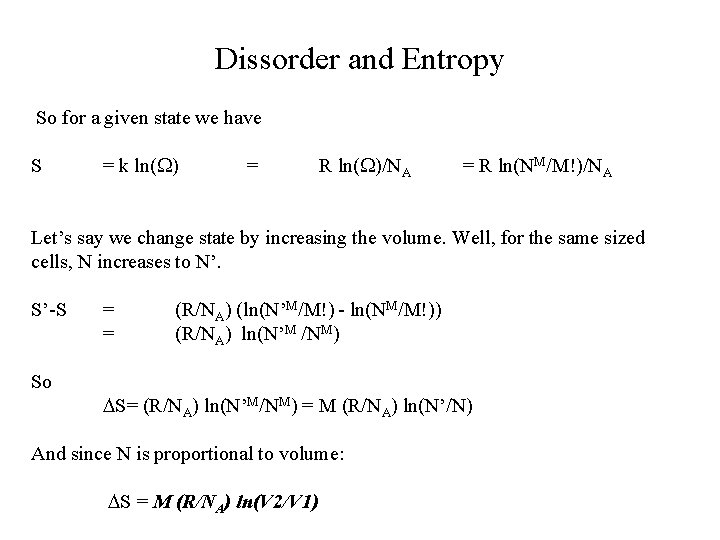Dissorder and Entropy So for a given state we have S = k ln( ) = R ln( )/NA = R ln(NM/M!)/NA Let’s say we change state by increasing the volume. Well, for the same sized cells, N increases to N’. S’-S So = = (R/NA) (ln(N’M/M!) - ln(NM/M!)) (R/NA) ln(N’M /NM) S= (R/NA) ln(N’M/NM) = M (R/NA) ln(N’/N) And since N is proportional to volume: S = M (R/NA) ln(V 2/V 1)Entropy of Materials Why does graphite have more entropy than diamond? Diamond S° 298= 2. 4 J/K How about water in its different phases: S° 298 (J/K mol) H 2 O(s, ice) H 2 O(l) H 2 O(g) 44. 3 69. 91 188. 72 Graphite S° 298= 5. 7 J/K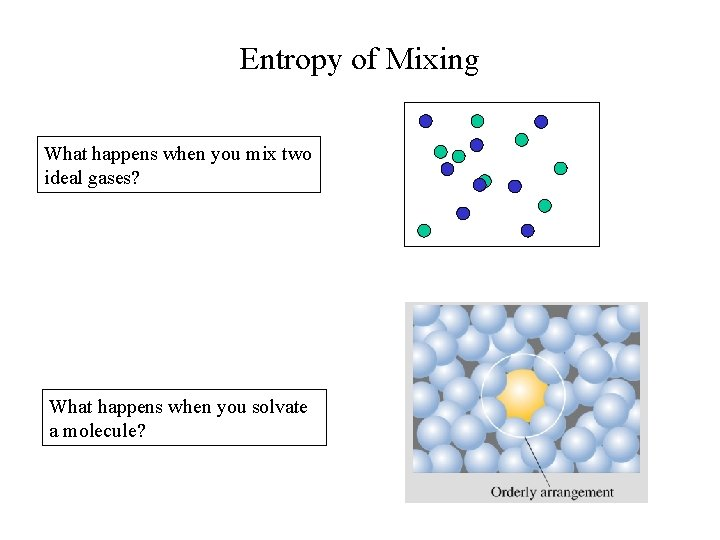Entropy of Mixing What happens when you mix two ideal gases? What happens when you solvate a molecule?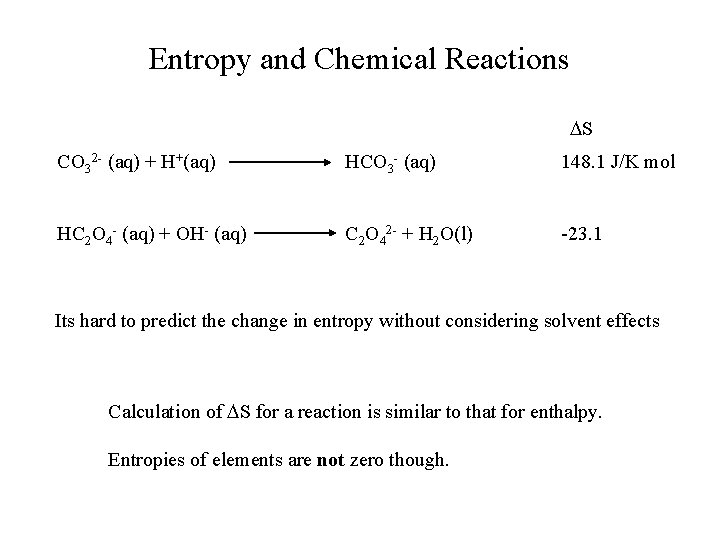Entropy and Chemical Reactions S CO 32 - (aq) + H+(aq) HCO 3 - (aq) 148. 1 J/K mol HC 2 O 4 - (aq) + OH- (aq) C 2 O 42 - + H 2 O(l) -23. 1 Its hard to predict the change in entropy without considering solvent effects Calculation of S for a reaction is similar to that for enthalpy. Entropies of elements are not zero though.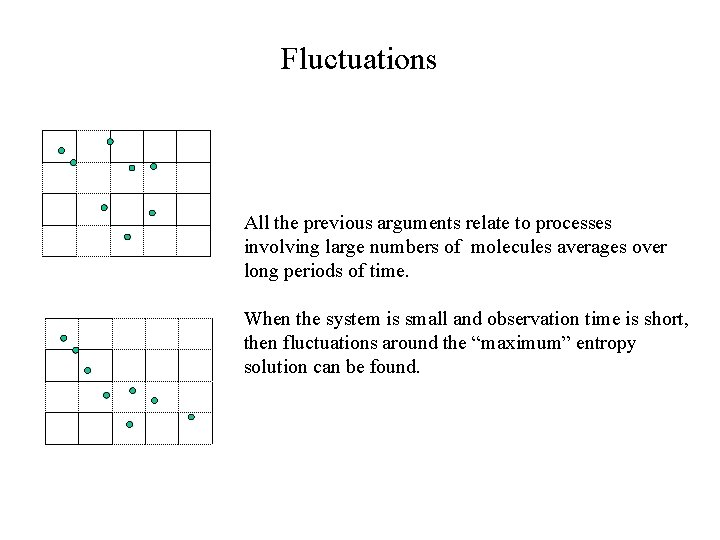Fluctuations All the previous arguments relate to processes involving large numbers of molecules averages over long periods of time. When the system is small and observation time is short, then fluctuations around the “maximum” entropy solution can be found.